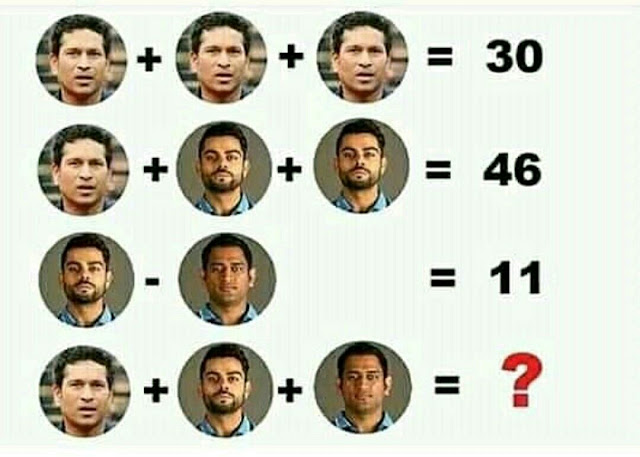# Sachin Kohli Dhoni Puzzle | with Answer

### Sachin Virat Dhoni Puzzle35

Explanation
Equation 1: 10 + 10 + 10 = 30 (Sachin = 10)
Equation 2: 10 + 18 + 18 = 46 (Kohli = 18)
Equation 3: 18 - 7 = 11 (Dhoni = 7)
Equation 4: 10 + 18 + 7 = 35